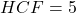## Two tankers contain 35 litres and 50 litres of petrol, the maximum capacity of a container which can measure the petrol of either tanker in

Question

Two tankers contain 35 litres and 50 litres of petrol, the maximum capacity of a container which can measure the petrol of either tanker in exact number of times : *

in progress 0
2 weeks 2021-08-29T00:09:23+00:00 1 Answers 0 views 0

The maximum capacity of a container which can measure the petrol of either tanker in exact number of times is 5 litres

Step-by-step explanation:

Given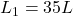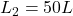To calculate the required maximum capacity, we simply calculate the HCF of both capacities.

We have:Factorize both: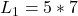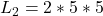The HCF is: